# Module 9: Linear Regression

The NHANES Tutorials are currently being reviewed and revised, and are subject to change. Specialized tutorials (e.g. Dietary, etc.) will be included in the future.

Linear Regression models, both simple and multiple, assess the association between independent variable(s) (Xi) — sometimes called exposure or predictor variables — and a continuous dependent variable (Y) — sometimes called the outcome or response variable. In cross-sectional surveys such as NHANES, linear regression analyses can be used to examine associations between covariates and health outcomes.

In cross-sectional surveys such as NHANES, linear regression analyses can be used to examine the association between multiple covariates and a health outcome. For example, we will assess the association between high density lipoprotein cholesterol (Y) and selected covariates (Xi) in this module. The covariates in this example will include race/ethnicity, age, sex, body mass index (BMI), smoking status, and education level.

You use simple linear regression when you have a single independent variable — and multiple linear regression when you have more than one independent variable (i.e., an exposure and one or more covariates). Multiple regression lets you understand the effect of the exposure of interest on the outcome after accounting for the effects of other variables (called covariates or confounders).

Simple linear regression is used to explore associations between one (continuous, ordinal or categorical) exposure and one (continuous) outcome variable. Simple linear regression lets you answer questions like, "How does HDL level vary with age?".

Multiple linear regression is used to explore associations between two or more exposure variables (which may be continuous, ordinal or categorical) and one (continuous) outcome variable. The purpose of multiple linear regression is to let you isolate the relationship between the exposure variable and the outcome variable from the effects of one or more other variables called covariates. For example, say that HDL levels tend to be higher among people with more income; and people with more income tend to be older. In this case, inferences about HDL and age get confused by the effect on HDL of income. This kind of "confusion" is called confounding (and these covariates are sometimes called confounders). Confounders are variables which are associated with both the exposure and outcome of interest. This relationship is shown in the following figure.

You can use multiple linear regression to see through confounding and isolate the relationship of interest. In this example, the relationship is between HDL cholesterol level and age. That is, multiple linear regression lets you answer the question, "How does HDL level vary with age, after accounting for — or unconfounded by — or independent of — income?" As mentioned, you can include many covariates at one time. The process of accounting for covariates is also called adjustment.

Comparing the results of simple and linear regressions can help to answer the question "How much did the covariates in the model distort the relationship between exposure and outcome (i.e., how much confounding was there)?"

Note that getting statistical packages like SUDAAN, SAS Survey, and Stata to run analyses is the easy part of regression. What is not easy is knowing which variables to include in your analyses, how to represent them, when to worry about confounding, determining if your models are any good and knowing how to interpret them. These tasks require thought, training, experience, and respect for the underlying assumptions of regression. Remember, garbage in - garbage out.

Finally, remember that NHANES analyses can only establish associations and not causal relationships. This is because the data are cross-sectional, so there is no way to establish temporal sequences (i.e., which came first the "exposure" or the "outcome"?).

This module will assess the association between high density lipoprotein cholesterol (the outcome variable) and selected covariates to show how to use linear regression with Stata. The covariates in this example will include race/ethnicity, age, sex, body mass index (BMI), smoking status, and education level. In other words, what is the effect of each of these variables, independent of the effect of the other variables?

Simple Linear Regression Model

In the simplest case, you plot the values of a dependent, continuous variable Y against an independent, continuous variable X1, (i.e. a correlation) and see the best-fit line that can be drawn through the points.

The first thing to do is make sure the relationship of interest is linear (since linear regression draws a straight line through data points). The best way to do this is to look at a scatterplot. If the relationship between variables is linear, continue (see panels a and b below). If it is not linear, do not use linear regression: Stata will draw a line, but that line won't adequately describe the data (see panels c and d below). In this case, you can try and transform the data or use other forms of regression such as polynomial regression.

Example of a Linear Relationship
Panel A Panel B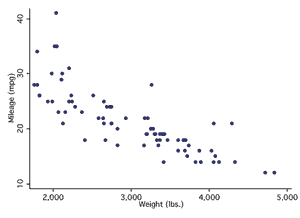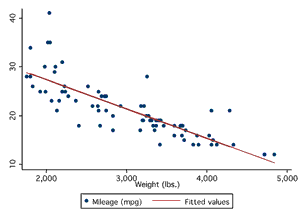Example of a Non-linear Relationship
Panel C Panel D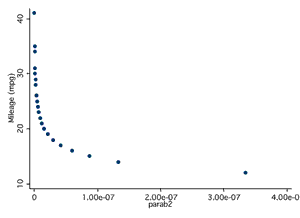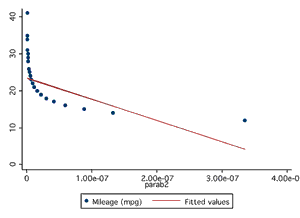This relationship between X1 and Y can be expressed as

b0 also known as the intercept, denotes the point at which the line intersects the vertical axis; b1 , or the slope, denotes the change in dependent variable, Y, per unit change in independent variable, X 1; and ε indicates the degree to which the plot of Y against X differs from a straight line. Note that for survey data, ε is always greater than 0.

Multiple Regression Model

You can further extend equation (1) to include any number of independent variables Xi , where i=1,..,n (both continuous (e.g. 0-100) and discrete (e.g. 0,1 or yes/no)).

The choice of variables to include in equation (2) can be based on results of univariate analyses, where Xi and Y have a demonstrated association. It also can be based on empirical evidence where a definitive association between Y and an independent variable has been demonstrated in previous studies.

Polynomial Regression

It is possible to have two continuous variables, Y and X1, on sampled individuals such that if the values of Y are plotted against the values of X1, the resulting plot would resemble a parabola (i.e., the value of Y could increase with increasing values of X, level off and then decline). A polynomial regression model is used to describe this relationship between X1 and Y and is expressed as

Interaction

Consider the situation described in equation (2), where a discrete independent variable, X2, and a continuous independent variable, X1, affect a continuous dependent variable, Y. This relationship would yield two straight lines, one showing the relationship between Y and X1 for X2=0, and the other showing the relationship of Y and X1 for X2=1. If these straight lines were parallel, the rate of change of Y per unit change in X1 would be the same for X2=0 as for X2=1, and therefore, there would be no interaction between X1 and X2. If the two lines were not parallel, the relationship between Y and X1 would depend upon the relationship between Y and X2, and therefore there would be an interaction between X1 and X2.

Interpretation of Coefficients

For continuous independent variables, the b coefficient indicates the change in the dependent variable per unit change in the independent variable, controlling for the confounding effects of the other independent variables in the model. A discrete random variable, X1, can assume 2 or more distinct values corresponding to the number of subgroups in a given category. For example, in the gender category there are 2 subgroups, men (Xi =1) and women (Xi = 2). One subgroup (usually arbitrarily) is designated as the reference group. The beta coefficient for a discrete variable indicates the difference in the dependent variable for one value of Xi , (e.g., the difference between women and the reference group, men), when all other independent variables in the model are held constant. A positive value for the beta coefficient indicates a larger value of the dependent variable for the subgroup (women) than for the reference group (men), whereas a negative value for the beta coefficient indicates a smaller value.

Interpretation of Coefficients Summary Table

Independent variable type Examples What does the b coefficient mean in Simple linear regression? What does the b coefficient mean in Multiple linear regression?
Continuous height, weight, LDL The change in the dependent variable per unit change in the independent variable. The change in the dependent variable per unit change in the independent variable after controlling for the confounding effects of the covariates in the model.
Categorical (also known as "discrete") sex (2 subgroups, men (sex =1) and women (sex = 2) where one is designated as the reference group (men, in this example). The difference in the dependent variable for one value of categorical variable (e.g., the difference between women and the reference group, men). The difference in the dependent variable for one value of categorical variable (e.g., between women and the reference group men), after controlling for the confounding effects of the covariates in the model.

SUDAAN ((proc regress), SAS Survey (proc survey reg), and Stata (svy:regress) procedures produce b coefficients, standard errors for these coefficients, confidence intervals, a t-statistic for the null hypothesis (i.e., b =0), a p-value for the t-statistic (i.e., the probability of obtaining a value greater than or equal to the value for the t statistic).

ANOVA Type Statistical Tests

In addition to the t-test, SUDAAN produces other test statistics with their corresponding p-values. These include the WALD F, Satterthwaite adjusted F, and Satterthwaite adjusted chi square statistics. SAS Survey procedures only produces the Wald F test with their corresponding p-values.

At the present time, the NHANES Analytic Guidelines do not make a recommendation about which statistic is the "best." Users are encouraged to frequently check the NHANES website for updated analytic guidelines. In the meantime, it is a good practice to examine all three statistics and the corresponding p-values for consistency. Users also are encouraged to compare the nominal degrees of freedom (i.e. the number of PSUs minus the number of strata containing observations) to the adjusted Satterthwaite degrees of freedom. Nominal degrees of freedom that are much larger than the adjusted Satterthwaite degrees of freedom may indicate model instability.

Generally speaking, the Satterthwaite adjusted F is the most conservative of the three statistics (i.e., it rejects the null hypothesis less often than do the other two statistics).

In this example, you will assess the association between high density lipoprotein (HDL) cholesterol — the outcome variable — and body mass index (bmxbmi) — the exposure variable — after controlling for selected covariates in NHANES 1999-2002. These covariates include gender (riagendr), race/ethnicity (ridreth1), age (ridageyr), smoking (smoker, derived from SMQ020 and SMQ040; smoker =1 if non-smoker, 2 if past smoker and 3 if current smoker) and education (dmdeduc).

Step 1: Specify the variables in the model

For continuous variables, you have a choice of using the variable in its original form (continuous) or changing it into a categorical variable (e.g. based on standard cutoffs, quartiles or common practice). The categorical variables should reflect the underlying distribution of the continuous variable and not create categories where there are only a few observations.

It is important to exam the data both ways, since the assumption that a dependent variable has a continuous relationship with the outcome may not be true. Looking at the categorical version of the variable will help you to know whether this assumption is true.

In this example, you could look at BMI as a continuous variable or convert it into a categorical variable based on standard BMI definitions of underweight, normal weight, overweight and obese. Here is how categorical BMI variables are created:

## IMPORTANT NOTE

These programs use variable formats listed in the Tutorial Formats page. You may need to format the variables in your dataset the same way to reproduce results presented in the tutorial.

Table of code to generate categorical BMI and eligibility variables
Code to generate categorical BMI variables Category
if 0 le bmxbmi lt 18.5 then bmicat= 1 ;
underweight
else if 18.5 le bmxbmi lt 25 then bmicat= 2 ;

normal weight

else if 25 le bmxbmi lt 30 then bmicat= 3 ;
overweight
else if bmxbmi ge 30 then bmicat= 4 ;
obese
if (lbdhdl^= . and riagendr^= . and ridreth1^= . and
ridageyr^=. and smoker^= . and dmdeduc^= <. and bmxbmi^= . )
and wtmec4yr>0 and (ridageyr>= 20)then eligible= 1 ;
eligibility

### Step 2: Create a simple linear regression

The association between the dependent and independent variables is expressed using the model statement in the in proc regress procedure. The dependent variable must be a continuous variable and will always appear on the left hand side of the equation. The variables on the right hand side of the equation are the independent variables and may be discrete or continuous.

Discrete variables are specified using a subgroup or a class statement. In proc regress, the dependent variable is NEVER specified in a subgroup or a class statement because it must be a continuous variable.

## IMPORTANT NOTE

These programs use variable formats listed in the Tutorial Formats page. You may need to format the variables in your dataset the same way to reproduce results presented in the tutorial.

Option 1. SUDAAN proc regress Procedure for Simple Linear Regression
Statements Explanation
proc sort data =analysis_data;by sdmvstra sdmvpsu; run ;
Use the proc sort procedure to sort the data by strata and primary sampling units (PSU) before running the procedure.
proc regress data=analysis_data;
Use the SUDAAN procedure, proc regress, to run multiple regression.
subpopn eligible=1 ;

Use the subpop eligible=1 statement to restrict the analysis to individuals with complete data for all the variables used in the final multiple regression model.

Because only those 20 years and older are of interest in this example, use the subpopn statement to select this subgroup. Please note that for accurate estimates, it is preferable to use subpopn in SUDAAN to select a subgroup for analysis, rather than select the study subgroup in the SAS program while preparing the data file.

nest sdmvstra sdmvpsu;
Use the nest statement to apply design-based methods of analysis.
weight wtmec4yr;
Use the weight statement to account for differential selection probabilities and to adjust for non-response. In this example, the examination weight for 4 years of data (wtmec4yr) is used. (For more information on how to select the correct weight for your analysis, see the Weighting module, Task 1.)
model lbdhdl= bmxbmi;
Use the model statement to define the associations to be assessed. Specify the dependent variable to the left-hand side of the equation and the independent variable on the right. This model will show the relationship between a unit increase in BMI and cholesterol level.
run ;
Option 2. SUDAAN proc regress Procedure for Simple Linear Regression with Categorical BMI Variable
Statements Explanation
proc regress data=analysis_data;
subpopn eligible=1 ;
nest sdmvstra sdmvpsu;
weight wtmec4yr;
model lbdhdl= bmicat;
run ;
Use the SUDAAN procedure, proc regress, to run multiple regression. This model will show the relationship between each unit increase in BMI category and cholesterol level.
Option 3. SUDAAN proc regress Procedure for Simple Linear Regression with Categorical BMI Variable and Reference Level
Statements Explanation
proc regress data=analysis_data;
subpopn eligible= 1 ;
nest sdmvstra sdmvpsu;
weight wtmec4yr;
class bmicat/nofreq;
reflevel bmicat=2 ;
model lbdhdl=bmicat;
rformat bmicat bmicat. ;
run ;
Use the SUDAAN procedure, proc regress, to run multiple regression. This model uses the normal BMI category as a reference category for cholesterol level.

Highlights from the output include:

• The results from the first model indicate that for each 1 unit increase of BMI, on average, HDL decreases by 0.69 mg/dl.
• The results from the second model indicate that, on average, HDL levels decrease by 5.6 mg/dl between the underweight BMI category and the normal weight BMI category, or the normal weight BMI category to the overweight BMI category.
• The results from the third model indicate that the relationship is not linear and the difference in HDL is between underweight and normal is 3.2 compared to a 7.5 difference between normal weight and overweight.

Step 3: Create a multiple regression

## IMPORTANT NOTE

These programs use variable formats listed in the Tutorial Formats page. You may need to format the variables in your dataset the same way to reproduce results presented in the tutorial.

SUDAAN proc regress Procedure for Multiple Linear Regression
Statements Explanation
proc sort data =analysis_data;
by sdmvstra sdmvpsu;
run ;
Use the proc sort procedure to sort the data by strata and primary sampling units (PSU) before running the procedure.
proc regress data=analysis_data;
Use the SUDAAN procedure, proc regress, to run multiple regression.
subpopn eligible=1;

Use the subpop eligible=1 statement to restrict the analysis to individuals with complete data for all the variables used in the final multiple regression model.

Because only those 20 years and older are of interest in this example, use the subpopn statement to select this subgroup. Please note that for accurate estimates, it is preferable to use subpopn in SUDAAN to select a subgroup for analysis, rather than select the study subgroup in the SAS program while preparing the data file.

nest sdmvstra sdmvpsu;
Use the nest statement to apply design-based methods of analysis.
weight wtmec4yr;
Use the weight statement to account for differential selection probabilities and to adjust for non-response. In this example, the examination weight for 4 years of data (wtmec4yr) is used. (For more information on how to select the correct weight for your analysis, see the Weighting module, Task 1.)
class riagendr ridreth1 smoker dmdeduc bmicat/nofreq;
Use the class statement to specify discrete variables. Note that any variables not specified in the class statement are treated as continuous. The dependent variable should NOT appear in the class statement. The nofreq option is used to suppress the printing of frequencies.
reflevel bmicat=2 ridreth1= 3 riagendr= 1 ;
Use the reflevel statement to change the reference level of a categorical variable. By default the reference level for a discrete variable is set to the last category. For bmicat this would be category 2 (normal weight). For ridreth1 this would be category 4 (Other race/ethnic groups). The reflevel statement changes the reference level to category 3 (non-Hispanic whites). For riagendr the default reference level is category 2 (females). This statement changes the reference level to category 1 (males).
model lbdhdl= riagendr ridreth1 ridageyr smoker dmdeduc bmicat;
Use the model statement to define the associations to be assessed. Specify the dependent variable to the left-hand side of the equation and the independent variables on the right.
effects smoker=( 1 - 1 0 )/ name= "Never smoker vs. past smoker" ;
Use the effects statement to test the hypothesis that HDL cholesterol for non-smokers is the same as that for past smokers.
lsmeans bmicat;
Use the lsmeans statement to produce means for the BMI categories (bmicat) and their standard errors. These means will be adjusted for age, smoking, gender, race/ethnicity, and education.
test waldf satadjf satadjchi;
Use the test statement to produce statistics and p-values for the Satterthwaite adjusted chi square (satadjchi), the Satterthwaite adjusted F (satadjf), and Satterthwaite adjusted degrees of freedom (printed by default). If this statement is omitted, the nominal degrees of freedom, the Wald F and the p-value corresponding to the Wald F and Wald P will be produced.

Step 4: Review Output and Highlights of the Results

In this step, the SUDAAN output is reviewed.

• HDL cholesterol is 6.55 mg/dL higher for overweight adults compared to normal weight adults, as defined by BMI.
• HDL cholesterol is 12.00 mg/dL higher for obese adults compared to normal weight adults, as defined by BMI.
• HDL cholesterol is 2.30 mg/dL lower for underweight adults compared to normal weight adults, as defined by BMI.
• HDL cholesterol is 9.98 mg/dL higher for women than for men, after adjusting for all other variables in the model.
• The F test for gender shows a significant effect (p < 0.001) of gender for HDL cholesterol when controlling for other covariates in the model.
• HDL cholesterol is 4.95 mg/dL higher for non-Hispanic Blacks compared to non-Hispanic Whites, after adjusting for all other variables in the model.
• HDL cholesterol increases 0.11 mg/dL per unit increase in age.

Special Topic: Interactions

When interactions are included in the model, they are denoted with an asterisk, *, between the two variables. An interaction can occur between a discrete and a continuous variable, or between two discrete variables. An interaction term will always appear on the right hand side of an equation.

See the sample code in Sample Datasets and Code for a model with interaction term included.

In this example, you will assess the association between high density lipoprotein (HDL) cholesterol and selected covariates in NHANES 1999-2002. These covariates include gender (riagendr), race/ethnicity (ridreth1), age (ridageyr), body mass index (bmxbmi), smoking (smoker, derived from SMQ020 and SMQ040; smoker =1 if non-smoker, 2 if past smoker and 3 if current smoker) and education (dmdeduc).

Step 1: Create Variable to Subset Population

In order to subset the data in SAS Survey Procedures, you will need to create a variable for the population of interest. You should not use a where clause or by-group processing in order to analyze a subpopulation with the SAS Survey Procedures.

In this example, restrict the analysis to individuals with complete data for all the variables used in the final multiple regression model. Then this variable is used in the domain statement to specify the population of interest.


if (LBDHDL^=. and RIAGENDR^=. and RIDRETH1^=. and SMOKER^=. and DMDEDUC^=. and BMXBMI^=.) and WTMEC4YR>0 and (RIDAGEYR>=20)
then ELIGIBLE=1; else ELIGIBLE=2;

Step 2: Recode Discrete Variables

To change the reference level for a discrete variable, recode the variable so that the desired reference category has the highest level.

The variable riagendr was recoded to make men the reference category. The name of the recoded variable is sex.

If RIAGENDR EQ 1 then SEX=2;
Else if RIAGENDR EQ 2 THEN SEX=1;

The variable ridreth1 was recoded to make non-Hispanic Whites the reference group. The recoded variable is ethn.

ETHN= RIDRETH1;
If RIDRETH1 eq 3 then ETHN=5;
Else if RIDRETH1 eq 4 then ETHN=2;
Else if RIDRETH1 eq 2 then ETHN=3;
Else if RIDRETH1 eq 3 then ETHN=4;

The variable bmicat was recoded to make normal weight the the reference group. The recoded variable is bmicatf.

if 0 le BMXBMI lt 18.5 then BMICATF=1;
else if 18.5 le BMXBMI lt 25 then BMICATF=4;
else if 25 le BMXBMI lt 30 then BMICATF=2;
else if BMXBMI ge 30 then BMICATF=3;

Step 3: Set up SAS Survey Procedures for Simple Linear Regression

The dependent variable should be a continuous variable and will always appear on the left hand side of the equation. The variables on the right hand side of the equation are the independent variables and may be discrete or continuous.

When interactions are included in the model, they are denoted with an asterisk, *, between the two variables. An interaction can occur between a discrete and a continuous variable, or between two discrete variables. An interaction term always will always appear on the right hand side of an equation.

The summary table below provides steps for performing linear regression analyses using SAS Survey procedures.

## IMPORTANT NOTE

These programs use variable formats listed in the Tutorial Formats page. You may need to format the variables in your dataset the same way to reproduce results presented in the tutorial.

Option 1. Use SAS Survey Procedures for Simple Linear Regression
Statements Explanation
PROC SURVEYREG DATA=analysis_data nomcar;
Use the SAS Survey procedure, proc surveyreg, to calculate significance. Use the nomcar option to read all observations.
STRATA sdmvstra;
Use the strata statement to specify the strata (sdmvstra) and account for design effects of stratification.
CLUSTER sdmvpsu;
Use the cluster statement to specify PSU (sdmvpsu) to account for design effects of clustering.
WEIGHT wtmec4yr;
Use the weight statement to account for the unequal probability of sampling and non-response. In this example, the MEC weight for 4 years of data (wtmec4yr) is used.
DOMAIN eligible;

Use the domain statement to restrict the analysis to individuals with complete data for all the variables used in the final multiple regression model.

## IMPORTANT NOTE

When using proc surveyreg, use a domain statement to select the population of interest. Do not use a where or by-group statement to analyze subpopulations with the SAS Survey Procedures.

MODEL lbdhdl= bmxbmi/CLPARM VADJUST=none;
Use a model statement to specify the dependent variable for HDL cholesterol (lbdhdl) as a function of the independent variable (BMI category). Body mass index (bmxbmi) is treated as continuous variable. The clparm option requests confidence limits for the parameters. The vadjust option specifies whether or not to use variance adjustment. This model will show the relationship between a unit increase in BMI and cholesterol level.
TITLE 'Linear regression model for high density lipoprotein and selected covariates: NHANES 1999-2002';

Use the title statement to label the output.

Option 2. Use SAS Survey Procedures for Simple Linear Regression with BMI Categorical Variable
Statements Explanation
PROC SURVEYREG
DATA analysis_data nomcar;
STRATA sdmvstra;
CLUSTER sdmvpsu;
WEIGHT wtmec4yr;
DOMAIN eligible;
TITLE'Linear regression model for high density lipoprotein and body mass index: NHANES 1999-2002' ;
Use the proc surveyreg procedure to perform linear regressions Use the nomcar option to read all observations. This model will show the relationship between each unit increase in BMI category and cholesterol level.
Option 3. Use SAS Survey Procedures for Simple Linear Regression with BMI Categorical Variable with Reference Level
Statements Explanation
PROC SURVEYREG
DATA analysis_data nomcar;
STRATA sdmvstra;
CLUSTER sdmvpsu;
WEIGHT wtmec4yr;
CLASS bmicatf;
DOMAIN eligible;
TITLE'Linear regression model for high density lipoprotein and body mass index: NHANES 1999-2002' ;

Use the proc surveyreg procedure to perform linear regression. Use the nomcar option to read all observations. This model uses the normal BMI category as a reference category for cholesterol level.

Use the class statement to denote the discrete variables included in the model; all other variables are treated as continuous. In this example, bmicatf is treated as a discrete variable.

Highlights from the output include:

• The results from the first model indicate that for each 1 unit increase of BMI, on average, HDL decreases by 0.69 mg/dl.
• The results from the second model indicate that, on average, HDL levels decrease by 5.6 mg/dl between the underweight BMI category and the normal weight BMI category, or the normal weight BMI category to the overweight BMI category.
• The results from the third model indicate that the relationship is not linear and the difference in HDL is between underweight and normal is 3.2 compared to a 7.5 difference between normal weight and overweight.

Step 4: Set Up SAS Survey Procedures for Multiple Linear

## IMPORTANT NOTE

These programs use variable formats listed in the Tutorial Formats page. You may need to format the variables in your dataset the same way to reproduce results presented in the tutorial.

Use SAS Survey Procedures for Multiple Linear Regression

Statements Explanation
PROC SURVEYREG DATA=analysis_data nomcar;
Use the SAS Survey procedure, proc surveyreg, to calculate significance. Use the nomcar option to read all observations.
STRATA sdmvstra;
Use the strata statement to specify the strata (sdmvstra) and account for design effects of stratification.
CLUSTER sdmvpsu;
Use the cluster statement to specify PSU (sdmvpsu) to account for design effects of clustering.
WEIGHT wtmec4yr;
Use the weight statement to account for the unequal probability of sampling and non-response. In this example, the MEC weight for 4 years of data (wtmec4yr) is used.
CLASS sex ethn smoker dmdeduc bmicatf;
Use the class statement to denote the discrete variables included in the model; all other variables are treated as continuous. In this example sex, ethnicity (ethn), smoking status (smoker), education (dmdeduc), and BMI (bmicatf) are treated as discrete variables.
DOMAIN eligible;

Use the domain statement to restrict the analysis to individuals with complete data for all the variables used in the final multiple regression model.

## IMPORTANT NOTE

When using proc surveyreg, use a domain statement to select the population of interest. Do not use a where or by-group statement to analyze subpopulations with the SAS Survey Procedures.

MODEL lbdhdl= sex ethn ridageyr dmdeduc smoker bmicatf/CLPARM vadjust=none;
Use a model statement to specify the dependent variable for HDL cholesterol (lbdhdl) as a function of the independent variable (BMI category). The clparm option requests confidence limits for the parameters. The vadjust option specifies whether or not to use variance adjustment. This model will show the relationship between BMI category and cholesterol level.
ESTIMATE 'Never vs past smoker' smoker 1 - 1 0 ;
Use the estimate statement to test for differences in HDL cholesterol between non-smokers and past smokers.
TITLE 'Linear regression model for high density lipoprotein and selected covariates: NHANES 1999-2002';
Use the title statement to label the output.

## IMPORTANT NOTE

SAS Survey Procedures proc surveyreg prints the Wald statistic and its p-value. It does not produce the Satterthwaite chi square or the Satterthwaite F statistics and their corresponding p-values. For these reasons, we recommend that you use proc regress in SUDAAN for multiple linear regression.

Step 5: Review Output and Highlights of the Results

In this step, the SAS Survey procedures output is reviewed.

• HDL cholesterol is 6.55 mg/dL higher for overweight adults compared to normal weight adults, as defined by BMI.
• HDL cholesterol is 12.00 mg/dL higher for obese adults compared to normal weight adults, as defined by BMI.
• HDL cholesterol is 2.30 mg/dL lower for underweight adults compared to normal weight adults, as defined by BMI.
• HDL cholesterol is 9.98 mg/dL higher for women than for men, after adjusting for all other variables in the model.
• HDL cholesterol is 4.95 mg/dL higher for non-Hispanic Blacks compared to non-Hispanic Whites, after adjusting for all other variables in the model.
• HDL cholesterol increases 0.11 mg/dL per unit increase in age.

In this example, you will assess the association between high density lipoprotein (HDL) cholesterol — the outcome variable — and body mass index (bmxbmi) — the exposure variable — after controlling for selected covariates in NHANES 1999-2002. These covariates include gender (riagendr), race/ethnicity (ridreth1), age (ridageyr), smoking (smoker, derived from SMQ020 and SMQ040; smoker =1 if non-smoker, 2 if past smoker and 3 if current smoker) and education (dmdeduc).

## IMPORTANT NOTE

There are several things you should be aware of while analyzing NHANES data with Stata. Please see the Stata Tips page to review them before continuing.

Step 1: Use svyset to define survey design variables

Remember that you need to define the SVYSET before using the SVY series of commands. The general format of this command is below:

svyset [w=weightvar], psu(psuvar) strata(stratavar) vce(linearized)

To define the survey design variables for your high density lipoprotein cholesterol analysis, use the weight variable for four-years of MEC data (wtmec4yr), the PSU variable (sdmvpsu), and strata variable (sdmvstra) .The vce option specifies the method for calculating the variance and the default is "linearized" which is Taylor linearization. Here is the svyset command for four years of MEC data:

svyset [w= wtmec4yr], psu(sdmvpsu) strata(sdmvstra) vce(linearized)

Step 2: Determine how to specify variables in the model

For continuous variables, you have a choice of using the variable in its original form (continuous) or changing it into a categorical variable (e.g. based on standard cutoffs, quartiles or common practice). The categorical variables should reflect the underlying distribution of the continuous variable and not create categories where there are only a few observations.

It is important to exam the data both ways, since the assumption that a dependent variable has a continuous relationship with the outcome may not be true. Looking at the categorical version of the variable will help you to know whether this assumption is true.

In this example, you could look at BMI as a continuous variable or convert it into a categorical variable based on standard BMI definitions of underweight, normal weight, overweight and obese. Here is how categorical BMI variables are created:

Table of code to generate categorical BMI variable
Code to generate categorical BMI variables BMI Category
gen bmicat=1 if bmxbmi>=0 & bmxbmi<18.5
underweight
replace bmicat=2 if bmxbmi>=18.5 & bmxbmi<25
normal weight
replace bmicat=3 if bmxbmi>=25
overweight
replace bmicat=4 if bmxbmi>=30 & bmxbmi<.
obese

Step 3: Determine the reference group for categorical variables

For all categorical variables, you need to decide which category to use as the reference group. If you do not specify the reference group options, Stata will choose the lowest numbered group by default.

Use the following general command to specify the reference group:

char var[omit]reference group value

For these analyses, use the following commands to specify the following reference groups.

Stata command Reference group
char ridreth1[omit]3
Non-Hispanic White
char smoker[omit]3
Current Smokers
char educ[omit]3
Greater than high school education
char bmicat[omit]2
Normal weight

Step 4: Create simple linear regression models to understand relationships

Before you perform a regression on the data, the data needs to meet a requirement — the dependent variable must be a continuous variable and the independent variables may be either discrete, ordinal, or continuous. The association between the dependent (or outcome) and independent (or exposure) variables is expressed using the svy:regress command. The general form of the command is:

svy:regress depvar indvar

Here is the command (and output) for the BMI-HDL example. This example uses the subpop (if eligible==1) statement to restrict the analysis to individuals with complete data for all the variables used in the final multiple regression model. The eligible variable is defined in the program available on the Sample Code and Datasets page.

svy, subpop(if eligible==1): regress lbdhdl bmxbmi

And, here is the output of the statement.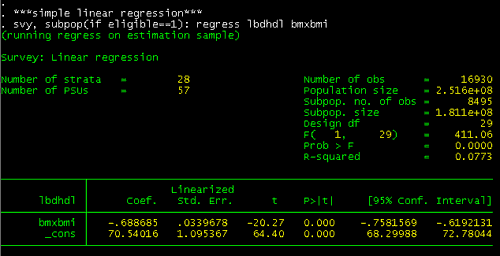This analysis says that for each 1 unit increase of BMI, on average, HDL decreases by 0.69 mg/dl. Or, you could do the simple regression using the BMI categories:

To perform the same analysis using the categorical BMI variable, bmicat, the statement would be:

svy, subpop(if eligible==1): regress lbdhdl bmicat

And, the output of that statement would be: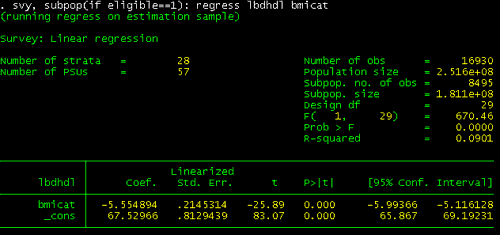This model says that, on average, HDL levels decrease by 5.6 mg/dl between the underweight BMI category and the normal weight BMI category, or the normal weight BMI category to the overweight BMI category.

Using the interaction expansion function (xi) to expand categorical variables into indicator variable sets

Delving deeper into this relationship, you will look at each comparison separately to see whether this continuous relationship really holds. Stata has a function (called xi or interaction expansion) which creates the "indicator variables" to allow you to see these relationships. The xi function will expand terms containing categorical variables (denoted i.varname) into indicator (also called dummy) variable sets. It has this general form:

xi:svy:regress depvar i.indvar

For this example, you will use the HDL variable as the dependent variable and the BMI categorical variable (bmicat) as the independent variable, denoted with the i. prefix. This example uses the subpop(if ridageyr >= 20 & ridageyr =.) statement to select participants who were age 20 years and older and did not have a missing value for the age variable.

xi:svy, subpop(if ridageyr >=20 & ridageyr =.): regress lbdhdl i.bmicat

Here are the results for this analysis which use "normal weight" - bmicat2 as the reference category: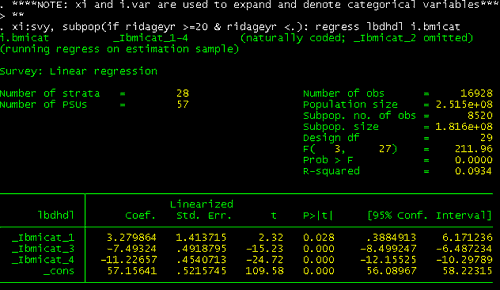This analysis using the BMI categorical variable (BMICAT) shows that the relationship is not linear and the difference in HDL is between underweight and normal is 3.2 compared to a 7.5 difference between normal weight and overweight.

## IMPORTANT NOTE

You can also just use the xi option to generate the indicator variables or interaction terms (rather than using it with the model command). The advantage of creating the indicators prior to the model, is that you do not need to write a command to set the reference category (you will do this implicitly by selecting the indicators you include in the model) and the output is easier to read (the xi model command repeats the individual components in interaction terms).

It is also possible to generate indicator variables using the tab, generate command:

tab var, gen(newvar); for example: tab bmicat, gen(ibmicat)

This command generates four variables: ibmicat1, ibmicat2, ibmicat3, and ibmicat4.

Step 5: Specify multiple linear regression models

Multiple linear regression uses the same command structure but now includes other independent variables. And if you want to create indicator variables for categorical variables, you will want to use the xi option. So, the general structure looks the same:

xi: svy: regress depvar indvar i.var

This example will use the HDL variable (lbdhdl) as the dependent variable. The independent categorical variables (riagendr, ridreth1, smoker, educ, and bmicat) are specified with the i. prefix, while ridageyr remains an independent continuous variable. Again, it uses the subpop(if ridageyr >= 20 & ridageyr =.) statement to select participants who were age 20 years and older and did not have a missing value for the age variable.

xi: svy, subpop(if ridageyr >=20 & ridageyr <.): regress lbdhdl i.riagendr i.ridreth1 ridageyr i.smoker i.educ i.bmicat

In this example, the output is: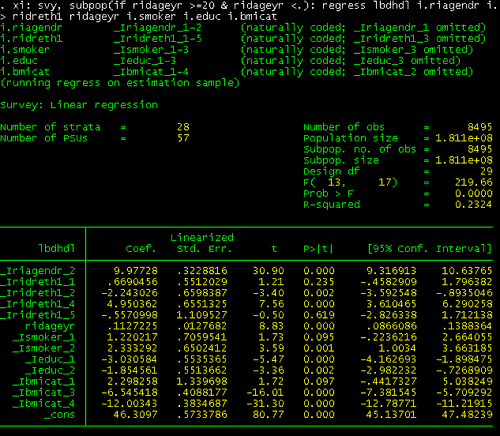Later in this module, the results of this multiple regression will be presented in a summary table comparing it with the univariate regression.

Step 6: Calculate means "adjusted" for the covariates in the model

Sometimes you may want to calculate means which are adjusted for the covariates specified in the model to allow you to see the effect of a given predictor variable. Stata has a built in command, adjust, to do this. Adjust is a post-estimation command

The adjust command uses only the sample mean, not the mean based on the survey design, when performing its computations. Therefore, if you want to use the survey mean, you would need to calculate it first and specify it explicitly in the adjust command. The following commands use summarize which is an rclass command and will not cause any trouble if run between the svy: regress and adjust commands; whereas svy:mean is an eclass command and cannot be used in between these commands. Here is the general form of the command:

sum _cat_1 [aw=weight] if conditions & e(sample)
local cat1 = r(mean)

...

adjust indvar1=cat1 indvar2=cat2.... if e(sample), by(indvar3)

The variables have to appear just as they are in the regression model. Independent categorical variables are specified with the _I prefix, while the independent continuous variable, ridageyr, doesn't not require the prefix. The following command, will generate mean HDL levels for BMI categories (by (bmicat)), adjusting for every other variable in the model.

sum _Ibmicat_1 [aw=wtmec4yr] if ridageyr >=20 & ridageyr <. & e(sample)
local bmicat1 = r(mean)
sum _Ibmicat_3 [aw=wtmec4yr] if ridageyr >=20 & ridageyr <. & e(sample)
local bmicat3 = r(mean)
sum _Ibmicat_4 [aw=wtmec4yr] if ridageyr >=20 & ridageyr <. & e(sample)
local bmicat4 = r(mean)

_Iridreth1_4='rid4' _Iridreth1_5='rid5' ridageyr='ridage' ///
_Ieduc_1='educ1' _Ieduc_2='educ2' _Ismoker_1='smoke1' ///
_Ismoker_2='smoke2' if ridageyr >=20 & ridageyr <. & e(sample), by(bmicat) se


The output for this example is: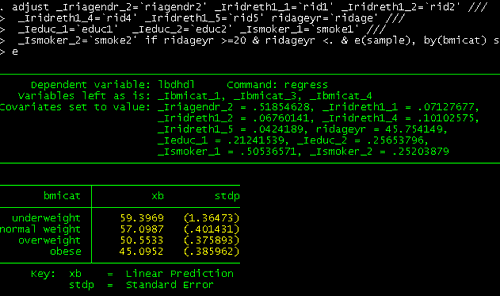Later in this module, the results of this adjusted means calculation will be presented in a summary table comparing it with the crude mean.

Step 7: Compare results of crude analysis (simple linear regression) and adjusted analysis (multiple linear regression)

To understand how much adjustment matters, it is helpful to compare the regression coefficient from the simple and multiple regression models. To help you review the results, the following summary tables present the crude analysis (simple linear regression) and adjusted analysis (multiple linear regression).

Table Comparing Differences between Crude Analysis (Simple Linear Regression) and Adjusted Analysis (Multiple Linear Regression) - BMI
BMI Crude Analysis
Mean HDL
Mean HDL
Crude Analysis
Coefficient*
(95% CI)
Coefficient*
(95% CI)
Crude Analysis
p value
p value
underweight 60.44 59.40 3.26
(.37 — 6.15)
2.30
(-.44 — 5.04)
.028 .097
normal 57.18 57.10 Reference
Group
Reference
Group
Reference
Group
Reference
Group
overweight 49.69 50.55 -7.48
(-8.50 — -6.46)
-6.55
(-7.38 — -5.71)
<.001 <.001
obese 45.94 45.10 -11.24
(-12.17 — -10.31)
-12.00
(-12.79 — -11.22)
<.001 <.001

Here are the summary tables of the results for the other covariates in the model.

Table Comparing Differences between Crude Analysis (Simple Linear Regression) and Adjusted Analysis (Multiple Linear Regression) - Smoking
Smoking Crude Analysis
Mean HDL
Mean HDL
Crude Analysis
Coefficient*
(95% CI)
Coefficient*
(95% CI)
Crude Analysis
p value
p value
current 49.35 51.27 Reference Group Reference Group
past 51.64 52.38 2.76
(1.29 — 4.23)
2.33
(1.00 — 3.66)
.001 .001
never 52 50.05 2.32
(.85 — 3.79)
1.22
(-.22 — 2.66)
.003 .095
Table Comparing Differences between Crude Analysis (Simple Linear Regression) and Adjusted Analysis (Multiple Linear Regression) - Sex
Sex Crude Analysis
Mean HDL
Mean HDL
Crude Analysis
Coefficient*
(95% CI)
(95% CI)
Crude Analysis
p value
p value
men 45.91 46.08 Ref erence Group Ref erence Group
women 56.21 56.06 10.30 (9.54 — 11.06) 9.98 (9.3 — 10.64) <.001 <.001
Table Comparing Differences between Crude Analysis (Simple Linear Regression) and Adjusted Analysis (Multiple Linear Regression) - Race/Ethnicity
Race/Ethnicity Crude Analysis
Mean HDL
Mean HDL
Crude Analysis
Coefficient*
(95% CI)
Coefficient*
(95% CI)
Crude Analysis
p value
p value
Non-Hispanic White 51.38 50.88 Reference Group Reference Group
Non-Hispanic Black 54.5 55.83 3.12
(1.54 — 4.70)
4.95
(3.61 — 6.29)
<.001 <.001
Other Hispanic 47.71 48.64 -3.67
(-5.47 — -1.88)
-2.24
(-3.59 — -.89)
<.001 .002
Mexican-American 48.92 51.55 -2.46
(-3.59 — -1.33)
.67
(-.46 — 1.80)
<.001 .235
Other 50.91 50.32 -.47
(-3.28 — 2.33)
-.56
(-2.83 — 1.71)
.733 .619
Table Comparing Differences between Crude Analysis (Simple Linear Regression) and Adjusted Analysis (Multiple Linear Regression) - Education
Education Crude Analysis
Mean HDL
Mean HDL
Crude Analysis
Coefficient*
(95% CI)
Coefficient*
(95% CI)
Crude Analysis
p value
p value
< high school 49.37 49.34 -3.10
(-4.41 — -1.79)
-3.03
(-4.16 — -1.90)
<.001 <.001>
high school 50.30 50.52 -2.18
(-3.23 — -1.12)
-1.85
(-2.98 — -.73)
<.001 .002
> high school 52.47 52.37 Reference Group Reference Group
Table Comparing Differences between Crude Analysis (Simple Linear Regression) and Adjusted Analysis (Multiple Linear Regression) - Age
Age Crude Analysis
Mean HDL
Mean HDL
Crude Analysis
Coefficient*
(95% CI)
Coefficient*
(95% CI)
Crude Analysis
p value
p value
Age
(years)
- - .11
(.07 — .12)
- <.001 <.001

Step 8: Perform post-estimation test

Use the test post estimation command to produce the Wald F statistic and the corresponding p-value. Use the nosvyadjust option to produce the unadjusted Wald F. In the example, the command test is used to test all coefficients together; all coefficients separately; and to test the hypothesis that HDL cholesterol for non-smokers is the same as that for past smokers.

The general form of this statement is below.

test indvar 1 ind var 2 ..., [nosvyadjust]

This example tests all of the coefficients.

test

Here are the results of that statement: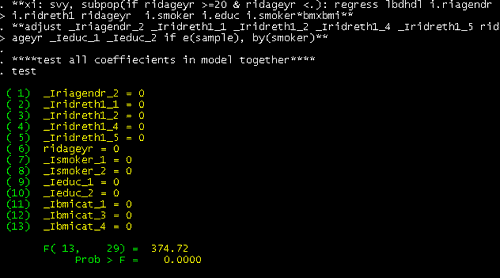The results of this test will be discussed in the next step.

This example tests the gender coefficient and, using the nosvyadjust option, produces the unadjusted Wald F. Tests for the additional variables are included in the program available on the Sample Downloads and Datasets page.

test _Iriagendr_2, nosvyadjust

Here are the results of that statement: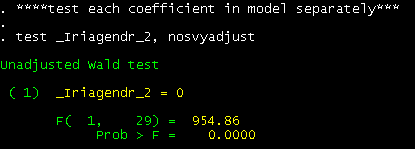The results of this test will be discussed in the next step.

This example tests the hypothesis that HDL cholesterol for non-smokers is the same as that for past smokers.

test _Ismoker_1 - _Ismoker_2 = 0

Here are the results of the statement: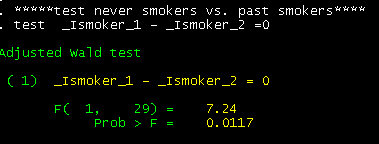The results of this test will be discussed in the next step.

Step 9: Review output

In this step, the Stata output is reviewed.

• HDL cholesterol is 6.55 mg/dL higher for overweight adults compared to normal weight adults, as defined by BMI.
• HDL cholesterol is 12.00 mg/dL higher for obese adults compared to normal weight adults, as defined by BMI.
• HDL cholesterol is 2.30 mg/dL lower for underweight adults compared to normal weight adults, as defined by BMI.
• HDL cholesterol is 9.98 mg/dL higher for women than for men, after adjusting for all other variables in the model.
• The F test for gender shows a significant effect (p < 0.001) of gender for HDL cholesterol when controlling for other covariates in the model.
• HDL cholesterol is 4.95 mg/dL higher for non-Hispanic Blacks compared to non-Hispanic Whites, after adjusting for all other variables in the model.
• HDL cholesterol increases 0.11 mg/dL per unit increase in age.
Stata svy:regres Command for Multiple Linear Regression
Statements Explanation
use "C:\Stata\tutorial\analysis_data.dta", clear

Use the use command to load the Stata-format dataset.

Use the clear option to replace any data in memory.

svyset sdmvpsu [pweight=wtmec4yr], strata(sdmvstra) vce(linearized)

Use the svyset command to declare the survey design for the dataset. Specifiy the psu variable sdmvpsu.

Use the [pweight=] option to account for the unequal probability of sampling and non-response. In this example, the MEC weight for four years of data (wtmec4yr) is used. Use the strata ( ) option to specify the stratum identifier (sdmvstra). Use the vce( ) option to specific the variance estimation method (linearized) for Taylor linearization.

char ridreth1[omit]3
char smoker[omit]3
char educ[omit]3
char bmicat[omit]2

Use these options to choose your reference group for the categorical variables. For example, the 3rd race/ethnicity (ridreth1) category (non-Hispanic White) is chosen as the reference group. If you do not specify the reference group options, Stata will choose the lowest numbered group by default.

xi: svy, subpop(if ridageyr >=20) vce(linearized): regress lbdhdl i.riagendr i.ridreth1 ridageyr bmxbmi i.smoker i.educ i.bmicat

Use the xi command to expand terms containing categorical variables (denoted i.varname) into indicator (also called dummy) variable sets. Use the svy: regress command to perform multiple linear regression to specify the dependent variable HDL cholesterol (lbdhdl) and independent variables, including: gender, race, age, body mass index, smoking, education and BMI category.

test
*******************************
test _Iridreth1_1 _Iridreth1_2 _Iridreth1_4 _Iridreth1_5, nosvyadjust
*******************************
test _Ismoker_1 - _Ismoker_2 =0

Use the test postestimation command to produce the Wald F statistic and the corresponding p-value. Use the nosvyadjust option to produce the unadjusted Wald F. In the example, the command test is used to test all coefficient together; all coefficients separately; and to test the hypothesis that HDL cholesterol for non-smokers is the same as that for past smokers.

Special topic: Interactions

If you want to look for interactions, use the xi option to create interaction terms. The general form for interaction terms is:

i.var1*i.var2

See the sample code in Sample Datasets and Code for a model with interaction term included.

Page last reviewed: 2/21/2020